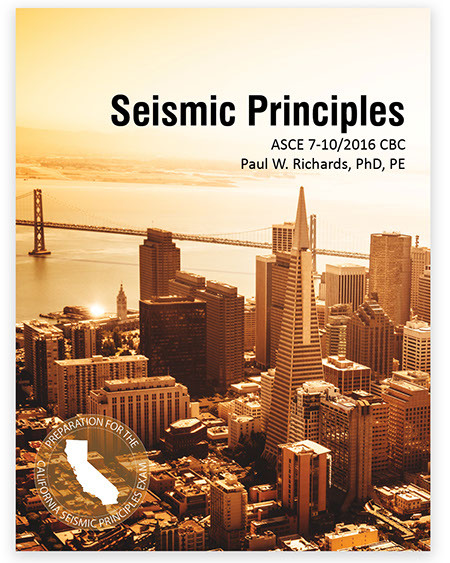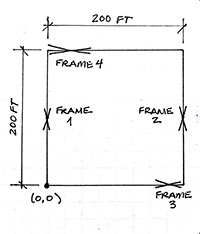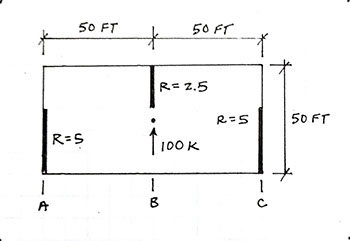Seismic Principles

21. A building in Seismic Design Category E has special reinforced concrete shear walls for the seismic force-resisting system. Which is true regarding the shear reinforcement for a wall that is 15 ft tall, 6 ft long, and 8 inches thick?

(a) One layer of shear reinforcement should be used.

(b) Two layers of shear reinforcement should be used.

(c) Shear reinforcement is not required.

(d) The number of layers does not matter, as long as the reinforcement ratio for the vertical bars is greater than 0.0025 and the bar spacing does not exceed 18 inches.

• Solution

Explanation

Requirements for special reinforced concrete shear walls are found in ACI 318 Section 18.10. It says that two layers of shear reinforcement are required if the required shear strength is above a certain threshold or when the height-to-length ratio exceeds 2. The wall in this problem has a height that is more than two times greater than its length, so two layers of shear reinforcement are required.

Did you miss this problem?

Many people do not have a current copy of ACI 318 and never learned about seismic detailing of concrete shear walls. Section 9.3 of Seismic Principles gives a concise overview of the detailing requirements for concrete shear walls and provides two fully-worked examples. The other sections in Chapter 9 discuss other reinforced concrete systems. Get your copy of the book.

22. A professional football stadium is being constructed on a site with SDS = 0.45g, SD1 = 0.15g, and Site Class B.  Are structural observations for seismic resistance required?

(a) No.

(b) Yes, and they must be performed by the engineer of record.

(c) Yes, but they can be performed by someone other than the engineer of record.

• Solution

Explanation

Section 1704.6.1 of the CBC lists the situations where structural observation for seismic resistance is required. This stadium would be in Seismic Design Category C because of the relatively low values for SDS and SD1, and structural observation for seismic resistance is not required.

Did you get this question wrong?

Section 13.4 of Seismic Principles explains the rules (and exceptions to the rules) pertaining to special inspections and structural observations. There are five fully-worked examples that address typical questions. Get your copy.

23. If a building has an importance factor of 1.5, which is true concerning the importance factors for nonstructural components within the building?

(a) All nonstructural components within the building will have an importance factor of 1.5.

(b) Nonstructural components that are necessary for safety or continued operation will have an importance factor of 1.5; other nonstructural components will have an importance factor of 1.25.

(c) Nonstructural components that are necessary for safety or continued operation will have an importance factor of 1.5; other nonstructural components will have an importance factor of 1.0.

(d) All nonstructural components will have an importance factor of 1.0Preview the Book

• Solution

Explanation

Nonstructural  components are addressed in Chapter 13 of ASCE 7. There are three situations where the component amplification factor is 1.5, otherwise it is 1.0. Section 11.2 of Seismic Principles explains these situations and provides three examples. Section 11.2 also explains which nonstructural components are exempt from seismic design requirements.

24. A particular story of a building has braced frames around the perimeter as shown below. Frames 1, 2, and 3 have the same stiffness. Frame 4 is twice as stiff as the other frames.Where is the center of rigidity?

(a) Cannot be determined

(b) (0,0)

(c) (100 ft, 100 ft)

(d) (100 ft, 133 ft)

• Solution

Explanation

Very few students learn anything about diaphragms as part of their undergraduate education. Chapter 5 of Seismic Principles will take you step-by-step through the procedures associated with rigid diaphragm analysis and design. Being able to determine the center of rigidity is a fundamental skill for rigid diaphragm analysis. Section 5.3 of Seismic Principles explains how to determine the center of rigidity and provides two fully-worked examples. Get your copy.

25. A 1-story building has a rigid diaphragms and the walls shown below. The rigidity, R, of each wall is indicated. A lateral force of 100 kips is distributed by the rigid diaphragm to the walls.How much of the 100 kips force is resisted by the wall on line A (left side)?

(a) 0 kips

(b) 5 kips

(c) 25 kips

(d) 40 kips

• Solution

Explanation

Rigid diaphragms distribute forces to walls in proportion to their stiffness (or rigidity). This problem is relatively easy because the walls are all oriented in the same direction and the center of rigidity and center of mass are aligned. Since the left wall has 40% of the total rigidity, it will resist 40% of the force, making (d) the best answer.

Most rigid diaphragm problems will have walls in both directions, and the center of rigidity offset from the center of mass. Such problems are significantly more complicated. Chapter 5 of Seismic Principles explains all the steps of rigid diaphragm analysis and has more than twenty full-worked examples. Get your copy.

26. The stability coefficient for the first story of a special moment frame is 0.11 (based on a first-order analysis). The maximum allowable coefficient value is 0.15. Which is true about the design?

(a) The design is permissible without considering P-Delta effects.

(b) The design is permissible if a second-order analysis is used.

(c) The design is permissible if there are no vertical irregularities.

(d) The design is not permissible.

• Solution

Explanation

The stability coefficient is calculated to determine if P-Delta effects are significant for a building. Section 12.8.7 of ASCE 7 provides the equation for the stability coefficient. If the stability coefficient is between 0.10 and the maximum allowable value, the design is permissible but P-Delta effects must be considered. Performing a second-order analysis is one way to satisfy the requirement.

Did you get this problem wrong?

Section 6.14 of Seismic Principles explains what P-delta effects are and provides two fully-worked examples on checking systems for P-delta effects. Get your copy of the book.

27. Which of the following can cause overstrength in buildings?

(a) Structural elements with sizes that are rounded-up from the minimum required for strength

(c) Strain hardening

(d) Material strengths greater than minimum assumed values

• Solution

Explanation

Load factors do not cause overstrength. Load factors are used in load combinations to determine the minimum required strength. Resistance factors, on the other hand, do contribute to overstrength because they penalize capacity for reasons that may not be valid for a particular building.

Did you get this problem wrong?

28. Loads used to compute diaphragm demands and loads used to compute demands on the walls/frames in a building are:

(a) The same, because the forces are transferred from the diaphragm to the walls/frames.

(b) The same, but the maximum force that occurs at any time during the earthquake should also be considered and may govern in the overall design.

(c) Possibly different, because the maximum diaphragm forces may occur at different points in time than the maximum base shear force.

(d) Possibly different, because some of the force in the diaphragm will be transferred to nonstructural elements of the building that are connected to it.

• Solution

Explanation

Diaphragm design loads are discussed in Section 12.10.1 of ASCE 7. Different formulas are used beyond the base shear formulas, so (c) and (d) are better answers than (a) and (b). Section 4.8 in Seismic Principles explains why the equations are different and provides a fully worked example. Get your copy.

29. A wood shear wall with blocking is 6 ft wide and 10 ft tall. The sheathing is 7/16 in. thick Structural 1. The edge nailing is 8d nails at 4 in. spacing.

What is the capacity of the wall for allowable stress design?

(a) 790 plf

(b) 395 plf

(c) 263 plf

(d) This wall is not permitted

• Solution

Explanation

Unit shear wall capacities are listed in Table 4.3A of SDPWS-AWC. The values read from Table 4.3A are divided by a safety factor of two to get a value for allowable stress design.

Did you get this question wrong?

Many people do not have a current version of SDPWS-AWC. Chapter 7 of Seismic Principles has a table with the same information as Table 4.3A. Example 7.5 in Seismic Principles demonstrates the solution to a problem that is a little more complicated than the one above. Get your copy.

30. A special masonry shear wall is 10 ft tall, 20 ft long, and has blocks laid in running bond.

What is the maximum spacing for the vertical reinforcement?

(a) 18 in.

(b) 24 in.

(c) 40 in.

(d) 48 in.

• Solution